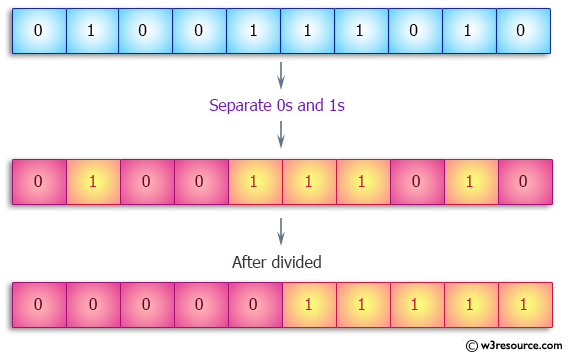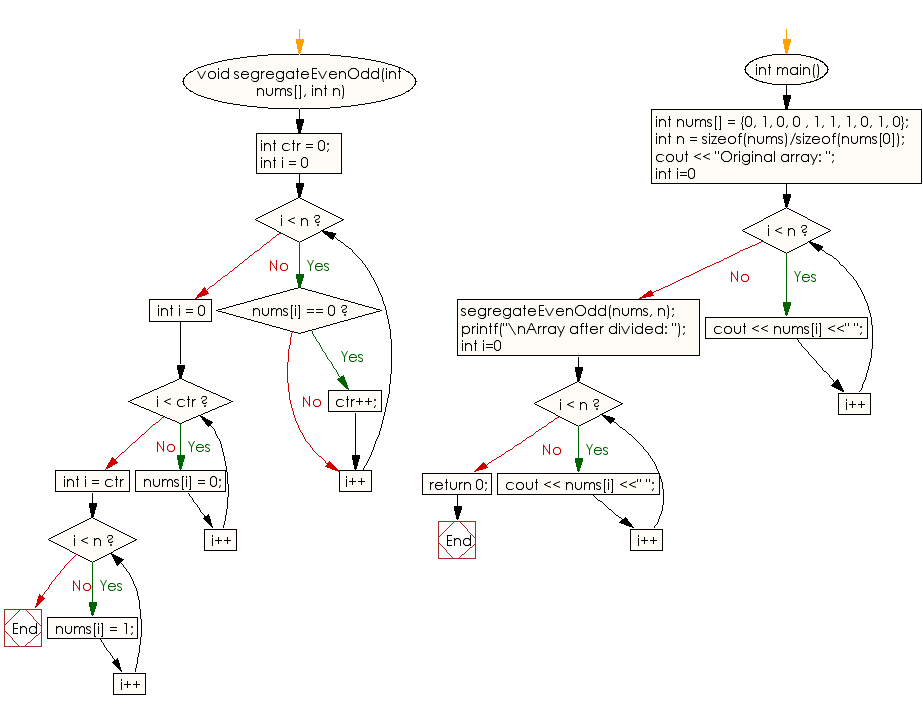﻿ C++ : Separate 0s and 1s from an array of values 0 and 1# C++ Exercises: Separate 0s and 1s from a given array of values 0 and 1

## C++ Array: Exercise-14 with Solution

Write a C++ program to separate 0s and 1s from a given array of values 0 and 1.

Pictorial Presentation:Sample Solution:

C++ Code :

``````#include<iostream>
using namespace std;

void segregateEvenOdd(int nums[], int n)
{
int ctr = 0;

for (int i = 0; i < n; i++) {
if (nums[i] == 0)
ctr++;
}

for (int i = 0; i < ctr; i++)
nums[i] = 0;

for (int i = ctr; i < n; i++)
nums[i] = 1;
}

int main()
{
int nums[] = {0, 1, 0, 0 , 1, 1, 1, 0, 1, 0};
int n = sizeof(nums)/sizeof(nums);
cout << "Original array: ";
for (int i=0; i < n; i++)
cout << nums[i] <<" ";
segregateEvenOdd(nums, n);

printf("\nArray after divided: ");
for (int i=0; i < n; i++)
cout << nums[i] <<" ";
return 0;
}
``````

Sample Output:

```Original array: 0 1 0 0 1 1 1 0 1 0
Array after divided: 0 0 0 0 0 1 1 1 1 1
```

Flowchart:C++ Code Editor:

What is the difficulty level of this exercise?

Test your Programming skills with w3resource's quiz.

﻿